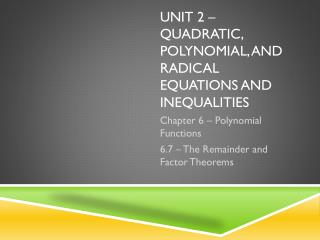Download Presentation# Unit 2 – quadratic, polynomial, and radical equations and inequalities - PowerPoint PPT Presentation

Download PresentationDownload Policy: Content on the Website is provided to you AS IS for your information and personal use and may not be sold / licensed / shared on other websites without getting consent from its author. While downloading, if for some reason you are not able to download a presentation, the publisher may have deleted the file from their server.

- - - - - - - - - - - - - - - - - - - - - - - - - - - E N D - - - - - - - - - - - - - - - - - - - - - - - - - - -
##### Presentation Transcript

1. Unit 2 – quadratic, polynomial, and radical equations and inequalities Chapter 6 – Polynomial Functions 6.7 – The Remainder and Factor Theorems

2. 6.7 – The Remainder and Factor Theorems • In this section we will learn how to: • Evaluate functions using synthetic substitution • Determine whether a binomial is a factor of a polynomial by using synthetic substitution

3. 6.7 – The Remainder and Factor Theorems • Synthetic Substitution • Synthetic division can be used to find the value of a function. Consider the polynomial function f(a) = 4a2 – 3a + 6. Divide the polynomial by a – 2. • Compare the remainder of 16 to f(2). f(2) = 4(2)2 – 3(2) + 6 = 16 – 6 + 6 = 16 *Notice that the value of f(2) is the same as the remainder when the polynomial is divided by a – 2. This illustrates the Remainder Theorem

4. 6.7 – The Remainder and Factor Theorems • Remainder Theorem • If a polynomial f(x) is divided by x – a, the remainder is the constant f(a), and • f(x) = q(x)  (x – a) + f(a) • dividend equals quotient times divisor plus remainder • where q(x) is a polynomial with degree one less than the degree of f(x)

5. 6.7 – The Remainder and Factor Theorems • When synthetic division is used to evaluate a function, it is called synthetic substitution. • It is a convenient way of finding the value of a function, especially when the degree of the polynomial is greater than 2.

6. 6.7 – The Remainder and Factor Theorems • Example 1 • If f(x) = 3x4 – 2x3 + x2 – 2, find f(4).

7. 6.7 – The Remainder and Factor Theorems • Factors of Polynomials • Synthetic division shows that the quotient of x4 + x3 – 17x2 – 20x + 32 and x – 4 is x3 + 5x2 + 3x – 8. • When you divide a polynomial by one of its binomial factors, the quotient is called a depressed polynomial. From the results of the division and by using the Remainder Theorem, we can make the following statement.

8. 6.7 – The Remainder and Factor Theorems • x4+ x3 – 17x2 – 20x + 32 = (x3 + 5x2 + 3x – 8)  (x – 4) + 0 • dividend equals quotient times divisor plus remainder • Since the remainder is 0, f(4) = 0. This means that x – 4 is a factor of x4 + x3 – 17x2 – 20x + 32. This illustrated the Factor Theorem, which is a special case of the Remainder Theorem.

9. 6.7 – The Remainder and Factor Theorems • Factor Theorem • The binomial x – a is a factor of the polynomial f(x) if and only if f(a) = 0. • If x – a is a factor of f(x), then f(a) has a factor of (a – a) or 0. Since a factor of f(a) is 0, f(a) = 0. • Now assume that f(a) = 0. If f(a) = 0, then the Remainder Theorem states that the remainder is 0 when f(x) is divided by x – a. This means that x – a is a factor of f(x). This proves the Factor Theorem.

10. 6.7 – The Remainder and Factor Theorems • Example 2 • Show that x – 3 is a factor of x3 + 4x2 – 15x – 18. Then find the remaining factors of the polynomial.

11. 6.7 – The Remainder and Factor Theorems • Example 3 • The volume of the rectangular prism is given by V(x) = x3 + 7x2 + 2x – 40. Find binomials with integer coefficients for the missing measures. x – 2

12. 6.7 – The Remainder and Factor Theorems HOMEWORK Page 359 #11 – 27odd, 28, 38 – 41